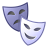# QlikView App Dev

Discussion Board for collaboration related to QlikView App Development.

Announcements
Join us at the Cloud Data and Analytics Tour! REGISTER TODAY
cancel
Showing results for
Search instead for
Did you mean:Not applicable

## Set Analysis Problem.

Hi There,

I really want to write this formula but not sure how...

I want all the entries for the current month

This works:

Sum({\$<KeyDate = {">=\$(=Only(AsAtMTD))<=\$(=Only(AsAtMonthEnd))"}>} BudgetNonAccommodationCleaningDailyHours)

But instead of AsAtMonthEnd I want this value which is the latest timesheet entry which is the result of the next expression.

Max({\$<KeyDate = {">=\$(=Only(AsAtMTD))<=\$(=Only(AsAtMonthEnd))"}, TimesheetHoursWorked={">0"}>} KeyDate)

How could I write the expression to achieve this?

e.g. Which doesn't work.

Sum({\$<KeyDate = {">=\$(=Only(AsAtMTD))"}, KeyDate = P({Max({\$<KeyDate = {">=\$(=Only(AsAtMTD))<=\$(=Only(AsAtMonthEnd))"}, TimesheetHoursWorked={">0"}>} KeyDate)>} )>} BudgetNonAccommodationCleaningDailyHours)

Thanks in advance

Luke

2 RepliesNot applicable
Author

Hi,

Store the value of this expression in a variable

vLatestTimesheetEntry = Max({\$<KeyDate = {">=\$(=Only(AsAtMTD))<=\$(=Only(AsAtMonthEnd))"}, TimesheetHoursWorked={">0"}>} KeyDate)

and then use this expression in set analysis

Sum({\$<KeyDate = {">=\$(=Only(AsAtMTD))<=\$(vLatestTimesheetEntry))"}>}Specialist II

Hi Like,

Sum({<KeyDate={">=\$(=Num(MonthStart(Max(KeyDate))))<=\$(=Max(KeyDate))"}, TimesheetHoursWorked={">0"} >} BudgetNonAccommodationCleaningDailyHours)

Hope this expression work for you.

-- Regards,

Vishal Waghole